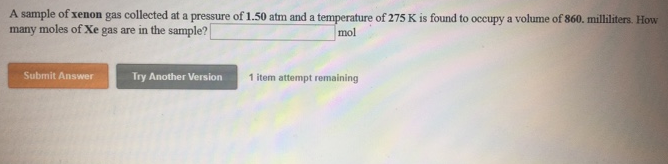# Problem: A sample of xenon gas collected at a pressure of 1.50 atm and a temperature of 275 K is found to occupy a volume of 860. Milliliters. How moles of Xe gas are in the sample?

###### FREE Expert Solution
81% (332 ratings)###### Problem Details

A sample of xenon gas collected at a pressure of 1.50 atm and a temperature of 275 K is found to occupy a volume of 860. Milliliters. How moles of Xe gas are in the sample?Frequently Asked Questions

What scientific concept do you need to know in order to solve this problem?

Our tutors have indicated that to solve this problem you will need to apply the The Ideal Gas Law concept. You can view video lessons to learn The Ideal Gas Law. Or if you need more The Ideal Gas Law practice, you can also practice The Ideal Gas Law practice problems.